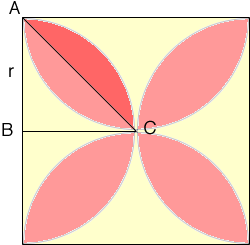SEARCH HOMEMath Central Quandaries & QueriesQuestion from James, a student: Four semi-circles are drawn inside a square, with the diameter being the length of the square.The overlapping portion of the semicircles are shaded. What fraction is shaded?Hi James,

I drew a diagram of your "flower", called the radius of the circle r and shaded half of one petal a darker pink.The area of the region shaded dark pink is the difference between the area of the sector ABC and the triangle ABC. The sector has a central angle of 90 degrees and a radius of r units and the triangle has a base of r units and a height of r units. The entire area of the flower is eight times this area.

I hope this helps,
PennyMath Central is supported by the University of Regina and The Pacific Institute for the Mathematical Sciences.